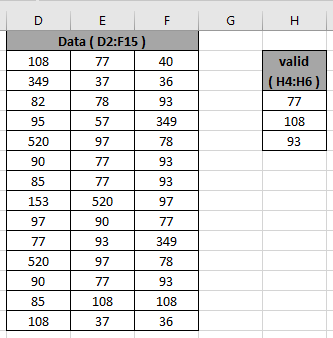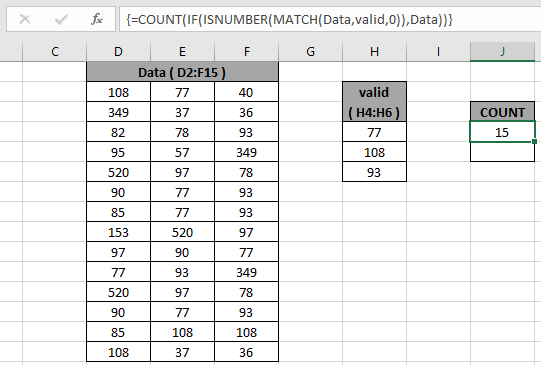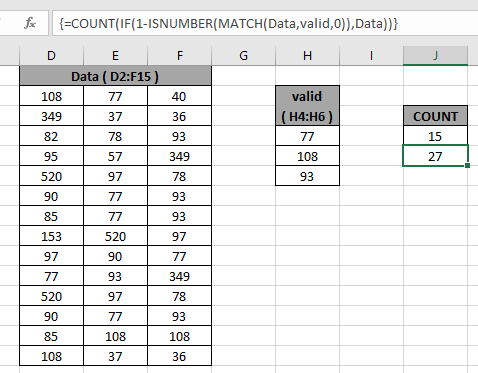# Count valid numbers in list

At Instance, we need to calculate the occurrence of some values which you require in the large list of values. Counting cells that have either of the valid numbers in excel explained here with an example.

For this article we will be needing the use the following functions:

We will construct a formula using the above functions. Here we need to first match the values and store the result in an array. Then array is filtered to get the matched values. Counting the results in the array gives the count of the valid numbers in a list or array.

Use the formula:

{ = COUNT ( IF ( ISNUMBER ( MATCH ( Data , valid , 0 ) ) , Data ) ) }

Variables:

Data - array or list where values needs to be matched

Valid - array or list of valid numbers to match.

Note: { } - Curly braces at the end & front of the formula must not be given manually, in fact it is given using the shortcut key Ctrl + Shift + Enter

Example:

Let’s understand this function using it in an example. Here we have a Data range from D2:F15 & a list of valid numbers from H4:H6 as shown in the snapshot below.We need to use the formula for the above stated problem.
Use the formula:

{ = COUNT ( IF ( ISNUMBER ( MATCH ( Data , valid , 0 ) ) , Data ) ) }

Explanation:

1. MATCH function matches the values in the list and return an array of #NA error and matched values as shown below.

= COUNT ( IF ( ISNUMBER(  { 2 , 1 , #N/A ; #N/A , #N/A , #N/A ; #N/A , #N/A , 3 ; #N/A , #N/A , #N/A ; #N/A , #N/A , #N/A ; #N/A , 1 , 3 ; #N/A , 1 , 3 ; #N/A , #N/A , #N/A ; #N/A , #N/A , 1 ; 1 , 3 , #N/A ; #N/A , #N/A , #N/A ; #N/A , 1 , 3 ; #N/A , 2 , 2 ; 2 , #N/A , #N/A } ) , Data))

1. ISNUMBER function returns TRUE for the numbers in array and FALSE for the errors in the array as shown below.

= COUNT ( IF  ( { TRUE , TRUE , FALSE ; FALSE , FALSE , FALSE ; FALSE , FALSE , TRUE ; FALSE , FALSE  , FALSE ; FALSE , FALSE , FALSE ; FALSE , TRUE , TRUE ; FALSE , TRUE , TRUE ; FALSE , FALSE , FALSE ; FALSE , FALSE , TRUE ; TRUE , TRUE , FALSE ; FALSE , FALSE , FALSE ; FALSE , TRUE ,TRUE ; FALSE , TRUE , TRUE ; TRUE , FALSE , FALSE } , Data ) )

1. IF function returns  an array of matched numbers and FALSE values.
2. COUNT Function counts the matched values in the list and returns its count.

Note: { } - Curly braces at the end & front of the formula must not be given manually, in fact it is given using the shortcut key Ctrl + Shift + EnterThe count of values in the list is 15. As you can see the formula counts the occurrence of values in the list.

You can exclude the values from the list and returns its count without considering values.
Use the formula:

{ = COUNT ( IF ( 1 - ISNUMBER ( MATCH ( Data , valid , 0 ) ) , Data ) ) }As you can see you can count both including values and excluding values from the list.

Here are some observational notes as mentioned below.

Notes:

1. Use the named range arrays carefully.
2. Curly braces are used for the function which accepts only one value and returns only one value but to get a range of values from the same functions we use Curly braces using the Ctrl + Shift + Enter.

Hope you understood how to Count valid numbers in list in Excel. Explore more articles on Excel Count functions here. Please feel free to state your query or feedback for the above article.

Related Articles

COUNTIFS with Dynamic Criteria Range : Count cells dependent on other cell values in Excel.

COUNTIFS Two Criteria Match : Count cells matching two different criteria on list in excel.

COUNTIFS With OR For Multiple Criteria : Count cells having multiple criteria match using the OR function.

The COUNTIFS Function in Excel : Count cells dependent on other cell values.

How to Use Countif in VBA in Microsoft Excel : Count cells using Visual Basic for Applications code.

How to use wildcards in excel : Count cells matching phrases using the wildcards in excel

Popular Articles

50 Excel Shortcut to Increase Your Productivity

Edit a dropdown list

If with conditional formatting

If with wildcards

Vlookup by date

Terms and Conditions of use

The applications/code on this site are distributed as is and without warranties or liability. In no event shall the owner of the copyrights, or the authors of the applications/code be liable for any loss of profit, any problems or any damage resulting from the use or evaluation of the applications/code.